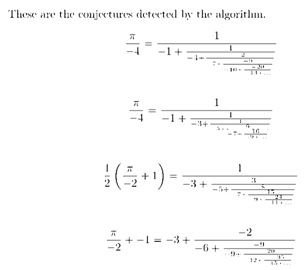# Ramanujan Machine

• Category
Science & Technology
• Published
1st Aug, 2019

Scientists from Technion — Israel Institute of Technology have developed a concept they have named the Ramanujan Machine, after the Indian mathematician.

### Context

Scientists from Technion — Israel Institute of Technology have developed a concept they have named the Ramanujan Machine, after the Indian mathematician.

• The Ramanujan 'machine' is, in reality, an algorithm. It attempts to automatically generate conjectures. Conjectures are mathematical statements which are proposed as true statements. In other words, they are mathematical statements that are, as yet, unproven.
• Typically, people provide the input and the algorithm finds the solution. For example, the user enters the ‘Destination’ in Google Maps and then the algorithm provides the user with the Navigation assistance.
• But, the Ramanujan Machine reverses the process. If a constant such as π is fed into the machine, it will generate a series whose value would lead towards π. Then, it is for the humans to prove that this proposed equation is correct.
• The researchers have also set up a website, ramanujanmachine.com. Users can suggest proofs for algorithms or propose new algorithms, which will be named after them.
• To date, the Ramanujan Machine focused on two algorithms, variants of Meet-In-The-Middle (MITM) algorithm and a Gradient Descent (GD).WHY IT IS NAMED ON RAMANUJAN?

• The algorithm reflects the way Srinivasa Ramanujan worked during his brief life (1887-1920). With very little formal training, he engaged with the most celebrated mathematicians of the time, particularly during his stay in England (1914-19), where he eventually became a Fellow of the Royal Society and earned a research degree from Cambridge.
• Throughout his life, Ramanujan came up with novel equations and identities —including equations leading to the value of pi — and it was usually left to formally trained mathematicians to prove these. In 1987, two Canadian brothers proved all 17 of Ramanujan’s series for 1/pi;
• Two years earlier, an American mathematician and programmer had used one of these formulas to calculate pi up to over 17 million digits, which was a world record at the time (Deka Baruah, Berndt & Chan; American Mathematical Monthly, 2009).

SIGNIFICANCE

• Conjectures are a major step in the process of making new discoveries in any branch of science, particularly mathematics. Equations defining the fundamental mathematical constants, including pi, are invariably elegant.
• It is quite possible that the algorithm will come up with conjectures that may take years to prove — a famous example of a human conjecture is Fermat’s Last Theorem, proposed in 1637 and proved only in 1994.

FAMOUS WORKS OF RAMANUJAN

• Srinivasa Ramanujan was one of India's greatest mathematical geniuses.
• He made substantial contributions to the analytical theory of numbers and worked on elliptic functions, continued fractions, and infinite series.
• Ramanujan was shown how to solve cubic equations in 1902 and he went on to find his own method to solve the quartic.
• He investigated the series ∑(1/n) and calculated Euler's constant to 15 decimal places.
• He began to study the Bernoulli numbers, although this was entirely his own independent discovery.
• He worked on Hyper-geometric series and investigated relations between integrals and series.
• After publication of a brilliant research paper on Bernoulli numbers in 1911 in the Journal of the Indian Mathematical Society he gained recognition for his work.
• Ramanujan worked out the Riemann series, the elliptic integrals, hypergeometric series and functional equations of the zeta function.
• Ramanujan left a number of unpublished notebooks filled with theorems that mathematicians have continued to study.

RAMANUJAN PRIZE

The ICTP Ramanujan Prize for Young Mathematicians from Developing Countries is a mathematics prize awarded annually by the International Centre for Theoretical Physics and named after the mathematician Srinivasa Ramanujan

SASTRA RAMANUJAN PRIZE

The SASTRA Ramanujan Prize, founded by Shanmugha Arts, Science, Technology & Research Academy (SASTRA) located near Kumbakonam, India, Srinivasa Ramanujan's hometown, is awarded every year to a young mathematician judged to have done outstanding work in Ramanujan's fields of interest.

HARDY–RAMANUJAN NUMBER

The number 1729 is known as the Hardy–Ramanujan number after a famous visit by Hardy to see Ramanujan at a hospital.